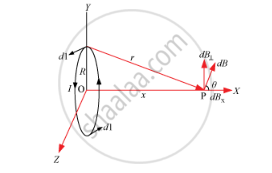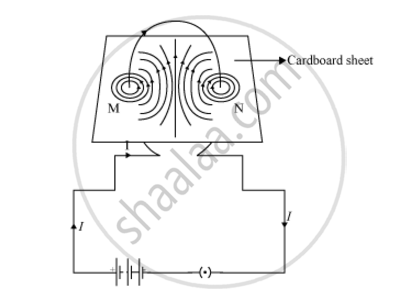# Use Biot-savart Law to Derive the Expression for the Magnetic Field on the Axis of a Current Carrying Circular Loop of Radius R. Draw the Magnetic Field Lines Due to a Circular Wire Carrying Current I. - Physics

Use Biot-Savart law to derive the expression for the magnetic field on the axis of a current carrying circular loop of radius R.

Draw the magnetic field lines due to a circular wire carrying current I.

#### SolutionI = Current in the loop

R = Radius of the loop

X-axis = Axis of the loop

X = Distance between O and P

dl = Conducting element of the loop

According to the BiotSavart law, the magnetic field at P is

dB=(mu_0)/(4pi) (I|dlxxr|)/r^3

r2 = x2 + R2

|dl × r| = rdl      (Because they are perpendicular)

:.dB=mu_0/(4pi) (Idl)/((x^2+R^2))

dB has two components: dBx and dB. dB is cancelled out and only the x-component remains.

dBx= dBcos θ

costheta= R/(x^2+R^2)^(1/2)

:.dB_x=(mu_0Idl)/(4pi) R/(x^2+R^2)^(3/2)

Summation of dl over the loop is given by 2πR.

:.B=B=B_xhati=(mu_0IR^2)/(2(x^2+R^2)^(3/2))hatiConcept: Magnetic Field on the Axis of a Circular Current Loop
Is there an error in this question or solution?